Question

What volume (in mL) of a 0.175 MHNO3 solution is required to completely react with 38.6 mL of a 0.100 MNa2CO3 solution according to the following balanced chemical equation?

Na2CO3(aq)+2HNO3(aq)?2NaNO3(aq)+CO2(g)+H2O(l)

V =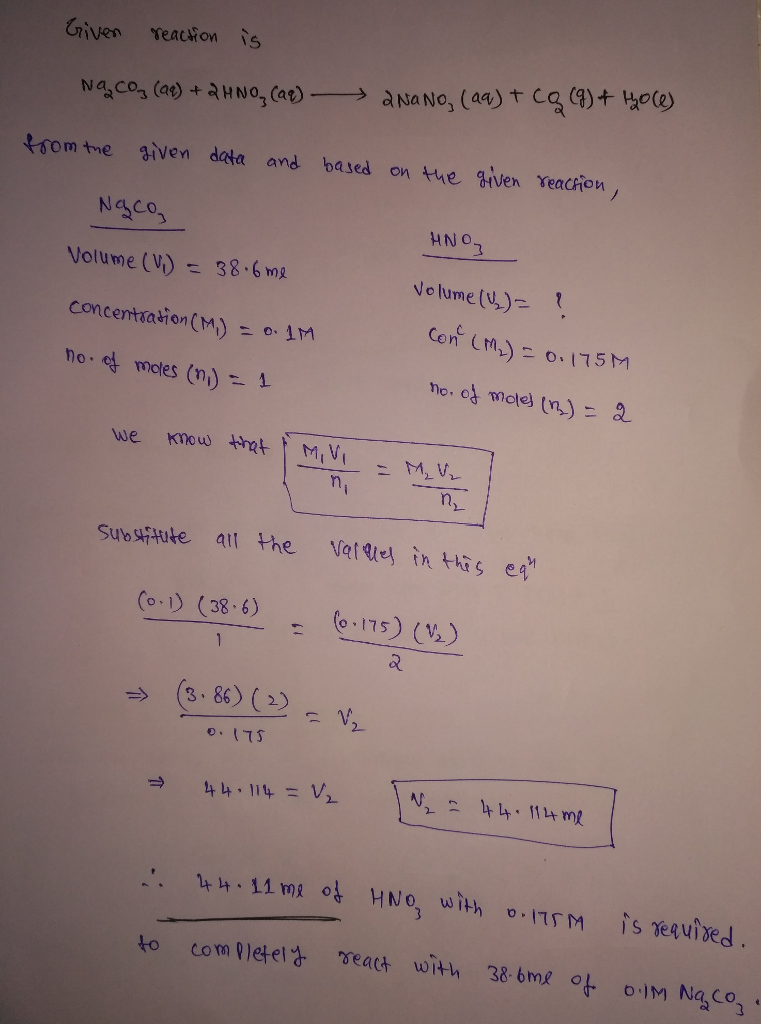#### Earn Coins

Coins can be redeemed for fabulous gifts.

Similar Homework Help Questions
• ### What volume (in mL) of a 0.220 M HNO3 solution will completely react with 39.0 mL...

What volume (in mL) of a 0.220 M HNO3 solution will completely react with 39.0 mL of a 0.125 M Na2CO3 solution according to this balanced chemical equation? Na2CO3(aq)+2 HNO3(aq)→2 NaNO3(aq)+CO2(g)+H2O(l)

• ### 1. How many milliliters of 0.112 M Na2CO3 will completely react with 27.2 mL of 0.135...

1. How many milliliters of 0.112 M Na2CO3 will completely react with 27.2 mL of 0.135 M HNO3 according to the reaction 2HNO3(aq) + Na2CO3(aq) → H2O(l) + CO2(g) + 2NaNO3(aq) Part B.) A 25.0-mL sample of HNO3 solution requires 35.7 mL of 0.108 M Na2CO3 to completely react with all of the HNO3 in the solution. What is the concentration of theHNO3 solution? Part C.)Consider the reaction HCl(aq) + NaOH(aq) → NaOH(aq) + H2O(l) How much 0.113 M NaOH...

• ### 1. What volume of 1.00 M HCl in liters is needed to react completely (with nothing...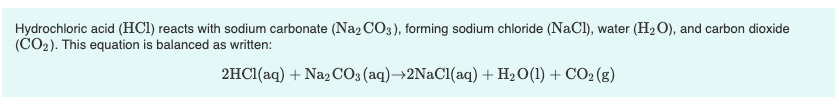1. What volume of 1.00 M HCl in liters is needed to react completely (with nothing left over) with 0.750 L of 0.200 M Na2CO3? Express your answer to three significant figures and include the appropriate units. 2. A 639-mL sample of unknown HCl solution reacts completely with Na2CO3 to form 15.1 g CO2. What was the concentration of the HCl solution? Express your answer to three significant figures and include the appropriate units. Hydrochloric acid (HCl) reacts with sodium...

• ### I need help Part A What volume (in mL) of a 0 200 M HNO, solution...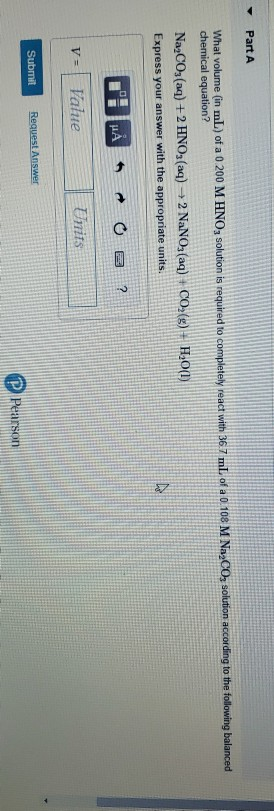I need help Part A What volume (in mL) of a 0 200 M HNO, solution is required to completely react with 36.7 mL of a 0.108 M NaaCO3 solution according to the following balanced chemical equation? NagCO3(aq) + 2 HNO3(aq) +2 NaNO3(aq) + CO2(g) + H2O(1) Express your answer with the appropriate units. THẢ5 0 3 ? V = Value Units Submit Request Answer P Pearson

• ### What volume (in L) of 0.300 M LiI will react with 0.150 L of a 0.175...

What volume (in L) of 0.300 M LiI will react with 0.150 L of a 0.175 M Pb(NO3)2 solution according to the following balanced chemical equation?                         2LiI(aq)   +     Pb(NO3)2(aq)    ⟶⟶ ⟶⟶ PbI2(s)    +   2LiNO3(aq)

• ### 8. What volume of a 5.00 M NaNO3 solution should you use to make 0.500 L...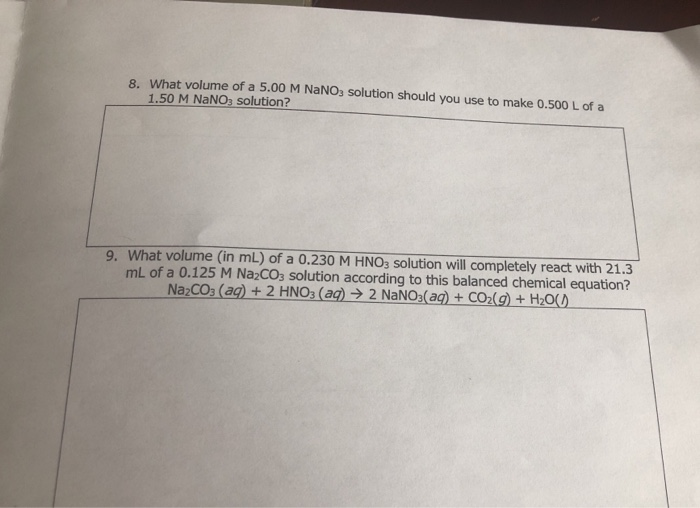8. What volume of a 5.00 M NaNO3 solution should you use to make 0.500 L of a 1.50 M NaNO3 solution? 9. What volume (in mL) of a 0.230 M HNO3 solution will completely react with 21.3 mL of a 0.125 M Na2CO3 solution according to this balanced chemical equation? NazCO3(aq) + 2 HNO3(aq) → 2 NaNO3(aq) + CO2(g) + H200)_

• ### Part A What volume of 1.00 M HCl in liters is needed to react completely (with...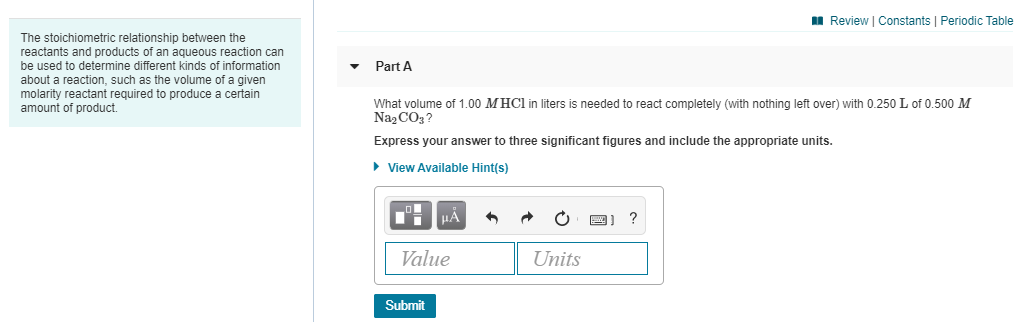Part A What volume of 1.00 M HCl in liters is needed to react completely (with nothing left over) with 0.250 L of 0.500 M Na2CO3? Express your answer to three significant figures and include the appropriate units. Part B A 419-mL sample of unknown HCl solution reacts completely with Na2CO3 to form 12.1 g CO2. What was the concentration of the HCl solution? Express your answer to three significant figures and include the appropriate units. A Review Constants Periodic...

• ### 3-What volume (in mL) of 0.125 M AgNO3 is required to react completely with 35.31 mL...

3-What volume (in mL) of 0.125 M AgNO3 is required to react completely with 35.31 mL of 0.225 M Na3 PO4? The balanced reaction is given below: 3 AgNO3(aq) + Nas PO4(aq) —>Ag3SO4(6)+ 3 NaNO3(aq) 4-What volume (in mL) of 0.550 M HCl is required to react completely with 4.72 g of sodium hydrogen carbonate? NaHCO3(s)+ HCl aq) —>NaCl(aq) + CO2(g)+ H2O) 5-Determine the volume of O2 (at STP) formed when 30.0 g of KCIO3 decomposes according to the following...

• ### What volume, in L, of 0.250 M KCl will completely react with 0.175 L of a...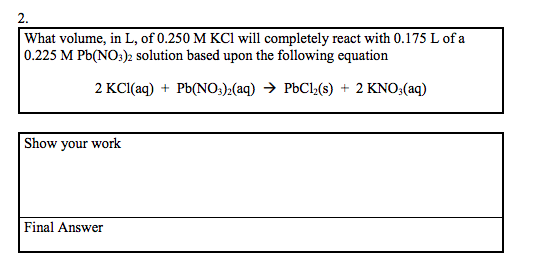What volume, in L, of 0.250 M KCl will completely react with 0.175 L of a 0.225 M Pb(NO3)2 solution based upon the following equation 2 KCl(aq) + Pb(NO3)2(aq) → PbCl2(s) + 2 KNO3(aq) Show your work Final Answer

• ### JUMPIUT 2 numerical question What volume (in L) of a 2.0 M HNO3 solution will completely...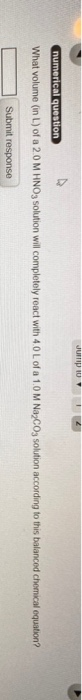JUMPIUT 2 numerical question What volume (in L) of a 2.0 M HNO3 solution will completely react with 4.0 L of a 1.0 M Na2CO3 solution according to this balanced chemical equation? Submit response